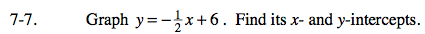Home > CAAC > Chapter 7 > Lesson 7.1.1 > Problem7-7

7-7.

Graph y =+ 6. Find its x- and y- intercepts. 7-7 HW eTool (Desmos). Homework Help ✎Remember the y-intercept is the point on the line where x = 0.
The x-intercept is the point on the line where y = 0.

Use the eTool below to help you solve this problem.
Click the link at right for the full version of the eTool: AC 7-7 HW eTool